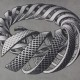# Definition: Disjoint Union

Definition: Let A and B be sets.  The disjoint union of A and B is the set, often denoted by$A\sqcup B$, of elements of the form$(a, A)$ or$(b, B)$ where$a\in A$ and$b\in B$.  We thus have, in symbols,$A\sqcup B=\{(a,A)|a\in A\}\cup \{(b,B)|b\in B\}$.//

The essence of this definition is to give us a way to take the union of sets while still “remembering” where each element came from, i.e., which set it’s from.  What this means is that even if the same element lies in both A and B, it will still form two distinct elements in the disjoint union because we’ve now “labeled” it with the set that it came from (by putting either A or B in the second slot according to which set the element is in originally).  This gives us, amongst other things, a perfectly logical way of creating sets that do “see duplicates”.

For more on this construction, see lesson 18.

Back to Glossary

Back to Lessons

### 2 Responses to Definition: Disjoint Union

1.Anonymous says:

What happens if A = B ?

•TrueBeautyOfMath says:

Good question! You then just basically get two copies of this set, one labeled by A, and one by B, so that the resulting set has twice as many elements as the original A and B.